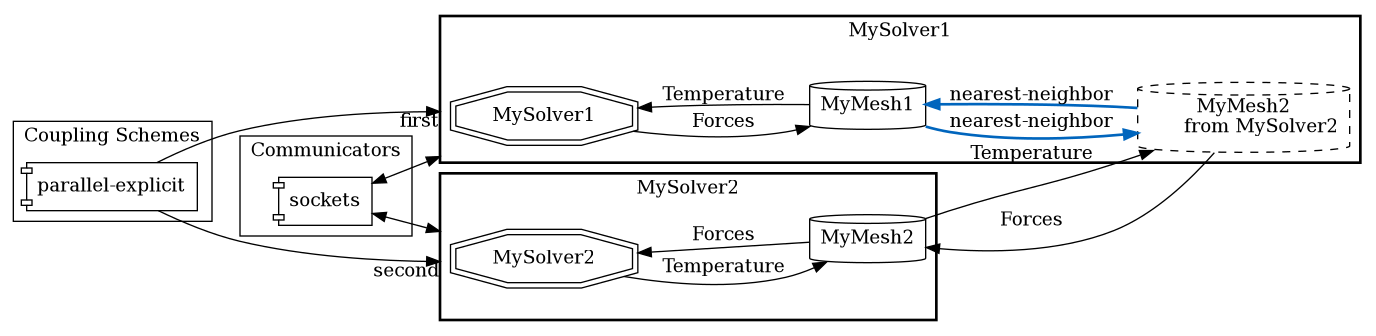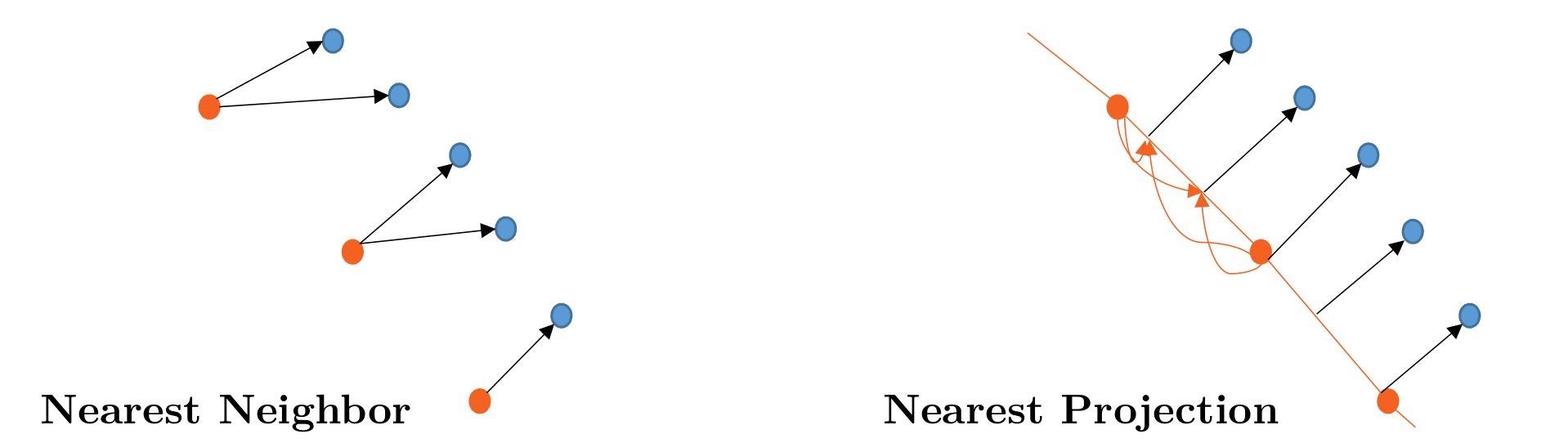When coupling two participants at a common coupling interface, in general, the two surface meshes do not match. Therefore, preCICE provides data mapping methods to map coupling data from one mesh to the other. On this page, we explain how to configure such data mapping methods.

## Basics

A participant needs to use at least two meshes to define a mapping between both:

<participant name="MySolver1">
<use-mesh name="MyMesh1" provide="yes"/>
<use-mesh name="MyMesh2" from="MySolver2"/>
<write-data name="Forces" mesh="MyMesh1"/>
<mapping:nearest-neighbor direction="write" from="MyMesh1" to="MyMesh2" constraint="conservative"/>
</participant>Mappings can be defined in two directions, either read or write:

• read mappings are executed before you can read data from the mesh. In the example above, Temperature is received on MyMesh2, then it is mapped from MyMesh2 to MyMesh1 and, finally, read from MyMesh1.
• write mappings are executed after you have written data.

Furthermore, mappings come int two types of constraint: consistent and conservative:

• conservative mapping: Mapping between different meshes (example, from a fine to a coarse grid), the value at a coarse node is computed as an aggregation of the corresponding fine nodes, such that the total coupling value (in our example Forces) on the coarse and fine mesh is the same. This is required for quantities that are absolute (extensive quantities, such as force, mass, etc.). An example for a nearest-neighbor mapping could look like this:
     f=2    f=1    f=2    f=1    f=1
------+------+------+------+------+------
\  |  /          |  /
-------------+-------------+-------------
f=(2+1+2)     f=(1+1)

• consistent mapping: For quantities that are normalized (intensive quantities; Temperature in our example, or pressure, which is force per unit area), we need a consistent mapping. This means that the value at coarse nodes is the same as the value at the corresponding fine node. Again, an example for a nearest-neighbor mapping could look like this:
     T=2    T=1    T=2    T=1    T=1
------+------+------+------+------+------
|             |
-------------+-------------+-------------
T=1           T=1


For a sequential participant, any combination of read/write-consistent/conservative is valid. For a parallel participant (i.e. a master tag is defined), only read-consistent and write-conservative is possible. More details are given further below.

Furthermore, mappings have an optional parameter timing, it can be:

• initial (the default): The mapping is only computed once, the first time it is used. This is sufficient for stationary meshes (also including the reference mesh in an Lagrangian or an ALE description).
• onadvance: The mapping is newly computed for every mapping of coupling data. This can be expensive and is only recommend if you know exactly why you want to do this.

Concerning mapping methods, preCICE offers four variants:

• nearest-neighbor: A first-order method, which is fast, easy to use, but, of course, has numerical deficiencies.
• nearest-projection: A (mostly) second-order method, which first projects onto mesh elements and, then, uses linear interpolation within each element (compare the figure below). The method is still relatively fast and numerically clearly superior to nearest-neighbor. The downside is that mesh connectivity information needs to be defined, i.e. in the adapter, the participant needs to tell preCICE about edges in 2D and edges, triangles, or quads (see issue) in 3D. On the mesh connectivity page, we explain how to do that. If no connectivity information is provided, nearest-projection falls back to an (expensive) nearest-neighbor mapping.
• linear-cell-interpolation: A second-order method similar to nearest-projection, but designed for volumetric coupling, where the coupling mesh is a region of space and not a domain boundary. It interpolates on triangles in 2D and on tetrahedra in 3D. If none are found, it falls back on nearest-projection.
• nearest-neighbor-gradient: A second-order method, which uses the same algorithm as nearest-neighbor with an additional linear approximation using gradient data. This method requires additional gradient data information. On the gradient data page, we explain how to add gradient data to the mesh. This method is only applicable with the consistent constraint.
• Radial-basis function mapping. Here, the configuration is more involved, so keep reading.Radial basis function mapping computes a global interpolant on one mesh, which is then evaluated at the other mesh. The global interpolant is formed by a linear combination of radially-symmetric basis functions centered on each vertex, enriched by one global linear polynomial. For more information, please refer, e.g., to Florian’s thesis (pages 37 ff.) or to this paper and the reference therein.

To compute the interpolant, a linear equation system needs to be solved in every mapping of data. We use either:

• the external library Eigen and a QR decomposition, or
• the external library PETSc and a GMRES solver.

For small/medium size problems, the QR decomposition is enough and you don’t need to install anything else. However, this follows a gather-scatter approach, which limits the scalability. For large problems, the GMRES solver performs better than the QR decomposition. For this, you need to build preCICE with PETSc. If you built with PETSc, the default is always GMRES. If you still want to use the QR decomposition, you can use the option use-qr-decomposition.

Radial basis function mapping also behaves as a second-order method just as nearest-projection, but without the need to define connectivity information. The downside is that it is normally more expensive to compute and that it shows numerical problems for large or highly irregular meshes.

The configuration might look like this:

<mapping:rbf-thin-plate-splines direction="read" from="MyMesh2" to="MyMesh1" constraint="consistent"/>


thin-plate-splines is the type of basis function used. preCICE offers basis function with global and local support:

• Basis function with global support (such as thin-plate-splines) are easier to configure as no further parameter needs to be set. For larger meshes, however, such functions lead to performance issues in terms of algorithmic complexity, numerical condition, and scalability.
• Basis functions with local support need either the definition of a support-radius (such as for rbf-compact-tps-c2) or a shape-parameter (such as for gaussian). To have a good trade-off between accuracy and efficiency, the support of each basis function should cover three to five vertices in every direction. You can use the tool rbfShape.py to get a good estimate of shape-parameter.

For a complete overview of all basis function, refer to this paper, page 5.

The interpolation problem might not be well-defined if you map along an axis-symmetric surface. This means, preCICE tries to compute, for example, a 3D interpolant out of 2D information. If so, preCICE throws an error RBF linear system has not converged or Interpolation matrix C is not invertible. In this case, you can restrict the interpolation problem by ignoring certain coordinates, e.g. x-dead="true" to ignore the x coordinate.

## Restrictions for parallel participants

As stated above, for parallel participants only read-consistent and write-conservative are valid combinations. If want to find out why, have a look at Benjamin’s thesis, page 85. But what to do if you want a write-consistent mapping? The trick is to move the mapping to the other participant, then write becomes read:

• Move the mapping, adjust write to read
• Be sure that the other participant also uses both meshes. Probably you need an additional <use-mesh name="MyMesh1" from="MySolver1"/>. This means another mesh is communicated at initialization, which can increase initialization time.
• Last, be sure to update the exchange tags in the coupling scheme, compare the coupling scheme configuration (e.g. change which mesh is used for the exchange and acceleration)

After applying these changes, you can use the preCICE Config Visualizer to visually validate your updated configuration file.

Maybe an example helps. You find one in the preCICE Forum.